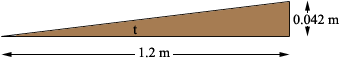SEARCH HOMEMath Central Quandaries & QueriesQuestion from Alan, a parent: how do I find the angle of an incline with a measurement of 0.042 with an adjacent of 1.2mtrs?. Thank YouAlan,

Is this the situation you have and you want to find the angle t?If so then tan(t) = 0.042/1.2 and you can use the inverse tangent function to find t.

PennyMath Central is supported by the University of Regina and The Pacific Institute for the Mathematical Sciences.